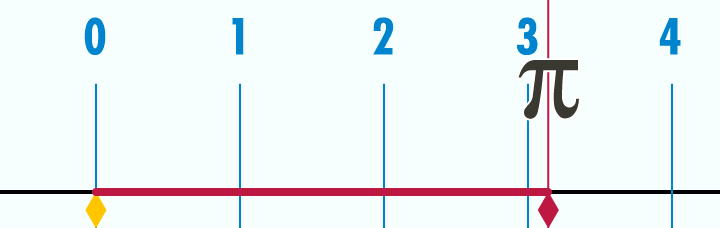## How to Calculate the Circumference of a Circle

In the previous post, we have learned about the basic terminologies about circles. We continue this series by understanding the meaning of circumference of a circle. The circumference of a circle is basically the distance around the circle itself. If you want to find the circumference of a can, for example, you can get a measuring tape and wrap around it.

The animation below shows, the meaning of circumference. As we can see, the circle with diameter 1 has circumference$\pi$ or approximately$3.14$.Note: If you want to know where$\pi$ came from, read Calculating the Value of Pi.

Example 1

What is the perimeter of a circle with diameter 1 unit?  » Read more

## Introduction to the Basic Concepts of Circles

The Civil Service Exams also contain geometry problems, and so far, our discussions are mostly algebra problems. In this new series of posts, we will discuss how to solve geometry and measurement problems particularly about circles. However, before we start solving problems, let us first discuss the basic terminologies about circles.

A circle is a set of points equidistant to a point called the center of the circle. As I go around to give trainings and lectures , I usually hear the wrong definition below. I am not sure where this definition originated, but this is wrong.  » Read more

## Introduction to the Concept of Perimeter

Aside from Algebra problems, the Civil Service Examination also contains geometric problems. In this post, I am going to introduce you to the concept of perimeter.

The perimeter of a polygon is the total distance around it. If you have a rectangular pool, for example, and you start walking from one corner along its edge (as near as you can), until you reach the place where you started, then the distance you have walked is its perimeter. » Read more